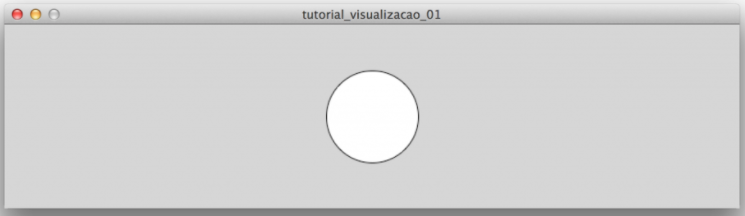Search

# Processing Assignment Help | Data Visualization Using Processing

In this blog we will learn how to use processing for data visualization, Processing language now has applications in several areas, including data visualization.Basic Commands

Run below command to draw basic command:

```size(1200, 200);
ellipse(600, 100, 100, 100);```

Output:These commands are called functions. We use two of them: size and ellipse.The first defines the size of the document we are going to work with (in pixels). The second draws an ellipse.

```size (width of the document, height of the document);
ellipse (horizontal coordinate of the ellipse, vertical coordinate of the ellipse, width of the ellipse, height of the ellipse);```

Look other commands:

```line()
point()
rect()
triangle()```

Visualization Using Data

Below the script which used to visualize the data

```size(1200, 200);
for(int i = 0;
i < tabela.getRowCount();
i = i + 1){
TableRow linha = tabela.getRow(i);
rect(120*i, 50, linha.getInt(“área”), linha.getInt(“área”));
}

```

Example to read text file data:

```// This code will print all the lines from the source text file.
println("There are " + lines.length + " lines.");
printArray(lines);```

Now draw the plot using csv data file

int[] data; void setup() { size(200, 200); // Load text file as a String String[] stuff = loadStrings("data.csv"); // Convert string into an array of integers using ',' as a delimiter data = int(split(stuff, ',')); } void draw() { background(255); stroke(0); for (int i = 0; i<data.length; i++) { // Use array of ints to set the color and height of each rectangle. rect(i*20, 0, 20, data[i]); } noLoop(); }If you need any programming assignment help in Processing Assignments, Processing project or Processing homework, then we are ready to help you.

Send your request at realcode4you@gmail.com and get instant help with an affordable price.

We are always focus to delivered unique or without plagiarism code which is written by our highly educated professional which provide well structured code within your given time frame.

If you are looking other programming language help like C, C++, Java, Python, PHP, Asp.Net, NodeJs, ReactJs, etc. with the different types of databases like MySQL, MongoDB, SQL Server, Oracle, etc. then also contact us.